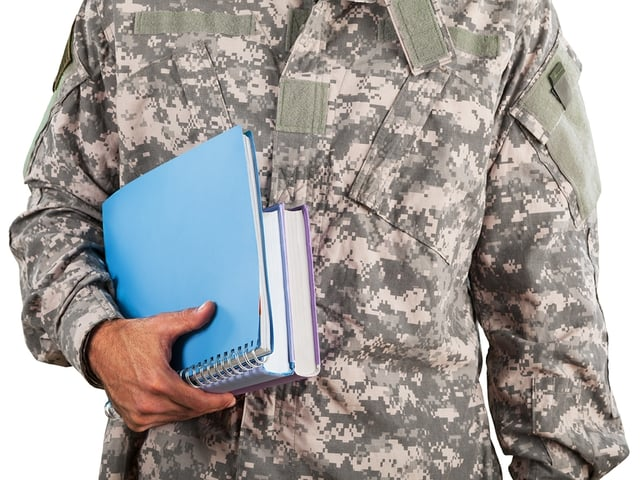# ASVAB Math Tests: What Do You Really Need to Know?

Test-takers commonly ask the question, “How much math do I need to know to pass the ASVAB?” The ASVAB is the Armed Forces Vocational Aptitude Battery. It assesses a person’s abilities to enlist in the U.S. Armed Forces and perform certain military or civilian jobs. The mathematics portion is broken into two sections: Arithmetic Reasoning and Mathematics Knowledge. The specific subtopics for these areas are listed at the end of this discussion, but here are some questions you may have and some related rough guidelines:

## Which ASVAB math section is harder?

You may have seen quite a few of the Arithmetic Reasoning topics in middle school or Pre-Algebra, but possibly not in enough depth, and you probably covered very little of the Mathematics Knowledge topics.

## Am I covered if I took Algebra I and II, plus Geometry?

If you took Algebra I and II and Geometry in high school, you probably covered everything on the test, but review the lists to be sure. Traditional Algebra I and II did not include statistics or probability, but many states and districts have rolled these topics into those courses. Otherwise, schools might offer statistics and probability in a separate course. Algebra I and II might have covered some of the Geometry as applied examples, but not included details like triangle types or parallel lines. The only Algebra II-specific topic is quadratic equations, but this may have been covered in sufficient depth in Algebra I. (You need to solve them, not graph them on this test.)

## What if I took “Integrated” or General Math classes?

“Integrated Math” or other general math classes may have covered much of the Arithmetic reasoning, especially the word problems and statistics, especially if you took 3 years of these classes. However, they may not have covered the Mathematics Knowledge topics.

## The ASVAB Arithmetic Reasoning Test includes:

• properties of numbers such as integer, rational irrational properties, and operations with negative numbers
• absolute value
• factors, multiples, and primes
• divisibility rules and remainders
• fractions and decimals
• exponents, radicals, scientific notation, including perfect squares and square roots
• factorials
• order of operations
• distributive, commutative properties
• ratio, proportion, and rates
• percentages
• average, mean, median, mode: the basics, such as determining these from a set of data or find a missing value given the mean
• probability: calculating based on outcomes, independent events, and random events
• sequences, arithmetic sequence
• word problems involving: rate, combined work problems, ratio and proportion, cross-multiplication, percent, and percent increase or decrease

The ASVAB Mathematics Knowledge Test includes all of the concepts listed under Arithmetic Reasoning, as well as these:

• monomials, binomials, algebraic expressions, and like terms
—multiplication: FOIL
—factoring: “unfoiling”
—binomial factoring patterns
• solving equations
• solving systems of equations
• quadratic equations: solve by factoring
• inequalities and plotting them on a number line
• lines, angles: right, acute, obtuse, vertical, and supplementary
• parallel lines and transversals